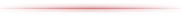### User ToolsCombo Score
Additional Amount = 8 Total = 8
Base Value = 0.7 Multiplication = x1 Total = 0.7

Combo Evaluation points will be added normally, and the rank will be D. The multiplier for the number of additional orbs accumulated will be the same.Combo Score
Additional Amount = 24+12 Total = 44
Base Value = 0.8 Multiplication = x2 Total = 2.3

Since Magma Drive hits just before an attack with straight charge, add 24 points for the “base value” and 12 points for the “follow up bonus”. Raises the rank to C and doubles the number of additional orbs accumulated.Combo Score
Additional Amount = 24 Total = 68
Base Value = 0.8 Multiplication = x5 Total = 6.3

If the added combo score is high, the rank may be skipped by one. The multiplier for the number of open additions is further increased.Combo Score
Additional Amount = 4 Total = 72